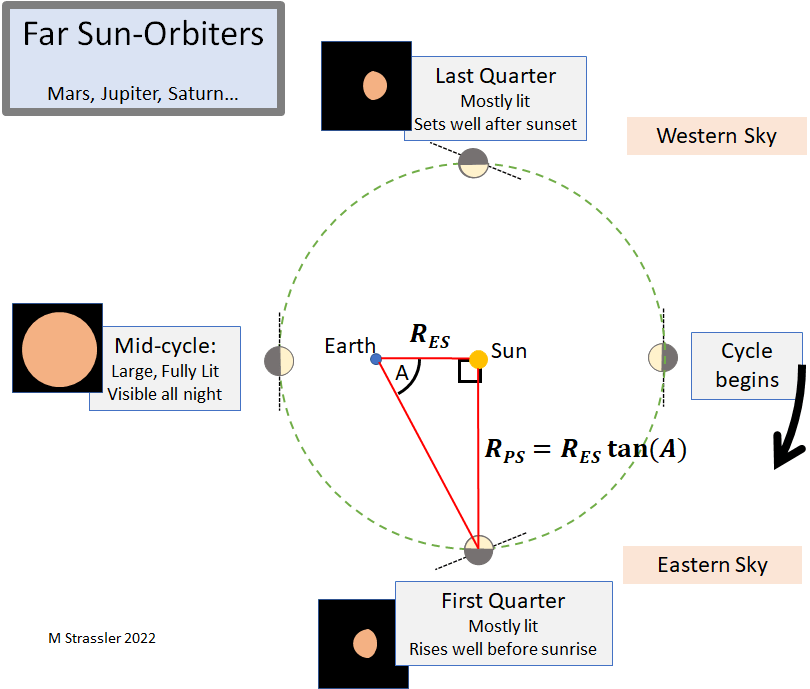## How to Estimate the Distance to the Outer Planets Yourself

Now, the last step in mapping out the other planets, before heading for more intriguing territory.

In a previous post I showed you how you can measure the distance between Venus and the Sun, RVS, relative to the distance between Earth and the Sun, RES. Under the assumption that Venus’s orbit around the Sun is circular (or nearly so), you can use the fact that when the angle between Venus and the Sun reaches its maximum (the moment of greatest elongation, and also approximately the moment when Venus appears half lit by the Sun), there’s a simple right-angle triangle in play. High school trigonometry then gives you the answer: RVS/RES ≈ 0.72 ≈ 1/√2. The same trick works for Mercury, which, like Venus, is a near Sun-orbiting planet, closer to the Sun than Earth.

But there’s no maximum angle for Mars, Jupiter, or the other far planets. These planets are further out than Earth and can even appear overhead at midnight, when they are 180 degrees away from the Sun. Fortunately there’s another right triangle we can use, again under the assumption of a (almost-)circular orbit, and that can give us a decent estimate.

### The Triangle for the Far Sun-Orbiting Planets

Let’s focus on Mars first, although the same technique will work on the outer planets. Mars has a cycle in which it disappears behind the Sun, from Earth’s perspective, on average every 780 days. (That start of the cycle is called “solar conjunction,” or just “conjunction” when the context is clear.) About half a cycle later, after on average 390 days, it is at “opposition”: closest to Earth, largest in a telescope, appearing overhead at midnight, and at its brightest. But if we wait only a quarter cycle, on average 195 days after conjunction, then the Mars-Sun line is at a 90 degree angle to the Earth-Sun line. That means that Mars, Earth and the Sun form a right-angle triangle with the right angle at the location of the Sun.

So on the day of first quarter we should measure the angle on the sky between Mars and the Sun. That’s the angle A on the figure below. Then the Mars-Sun distance RMS and the Earth-Sun distance RES are the two sides of a right-angle triangle. That means they are related by the tangent function:

• RMS/RES = tan A.For a planet whose distance RPS from the Sun is greater than Earth’s, an estimate of its distance compared to the Earth-Sun distance RES can be made using the fact that (were its orbit perfectly circular) it would make a right-angle triangle after the first or third quarter of its cycle, with the right angle located at the Sun. Sizes of Earth, Sun and planet not shown to scale.